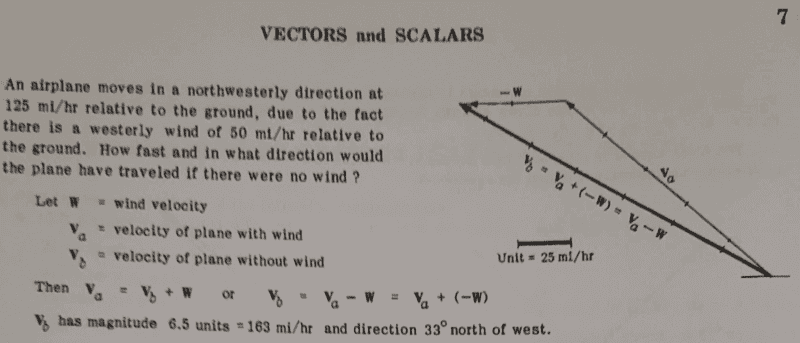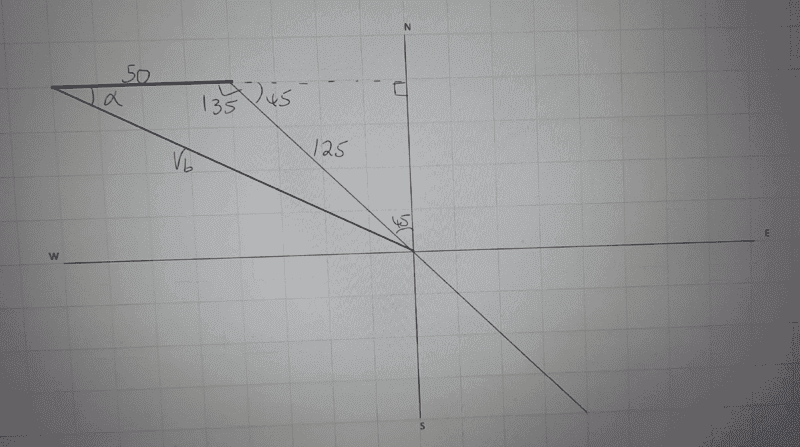# Find the velocity of the airplane if there was no wind

Gold Member
Homework Statement:
See attached
Relevant Equations:
Understanding of vectors
Find the question and its solution below;Ok i realized that we could also use cosine rule here, in my approach i considered the sketch below;##V_b= 18,125- (2×50×125×cos 135)##
##V_b=164.2##

To find direction, i used sine rule;
##\frac {125}{sin α}##=##\frac {164.2}{sin 135}##
##α=32.56##

Would this be correct? ...i can see there is a small difference on the value of the magnitude.